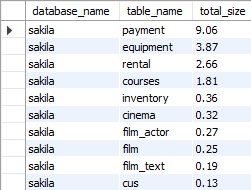## List 10 largest tables in MySQL database

Article for:

This query returns list of ten largest (by data size) tables.

## Query

``````select table_schema as database_name,
table_name,
round( (data_length + index_length) / 1024 / 1024, 2)  as total_size,
round( (data_length) / 1024 / 1024, 2)  as data_size,
round( (index_length) / 1024 / 1024, 2)  as index_size
from information_schema.tables
where table_schema not in ('information_schema', 'mysql',
'performance_schema' ,'sys')
and table_type = 'BASE TABLE'
-- and table_schema = 'your database name'
order by total_size desc
limit 10;
``````

## Columns

• database_name - table's schema name
• table_name - table name
• total_size - total table size
• data_size - size of table's rows
• index_size - size of indexes

## Rows

• One row represents one table
• Scope of rows: ten tables with the biggest total size
• Ordered by total, data and index size

## Sample results0